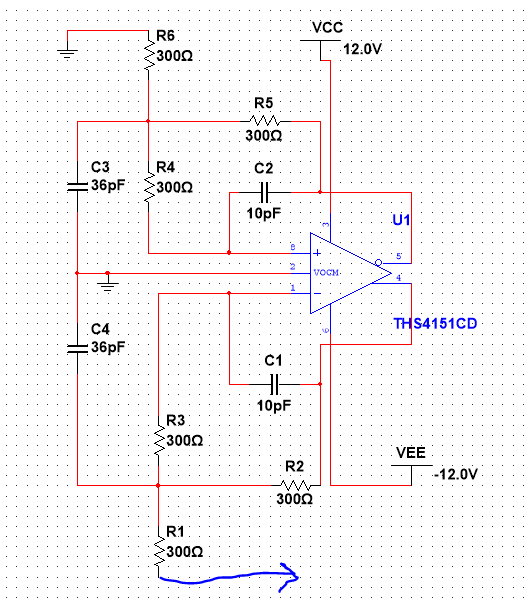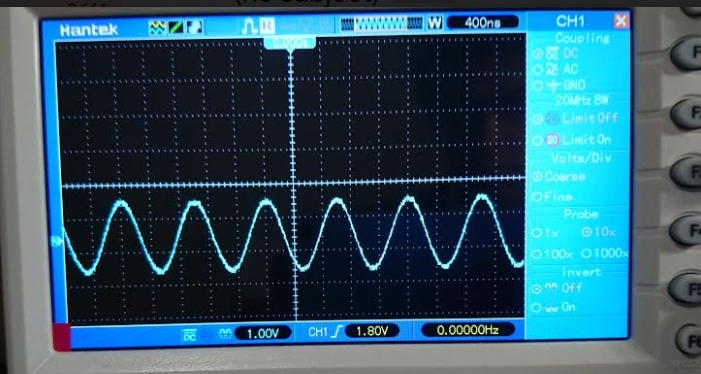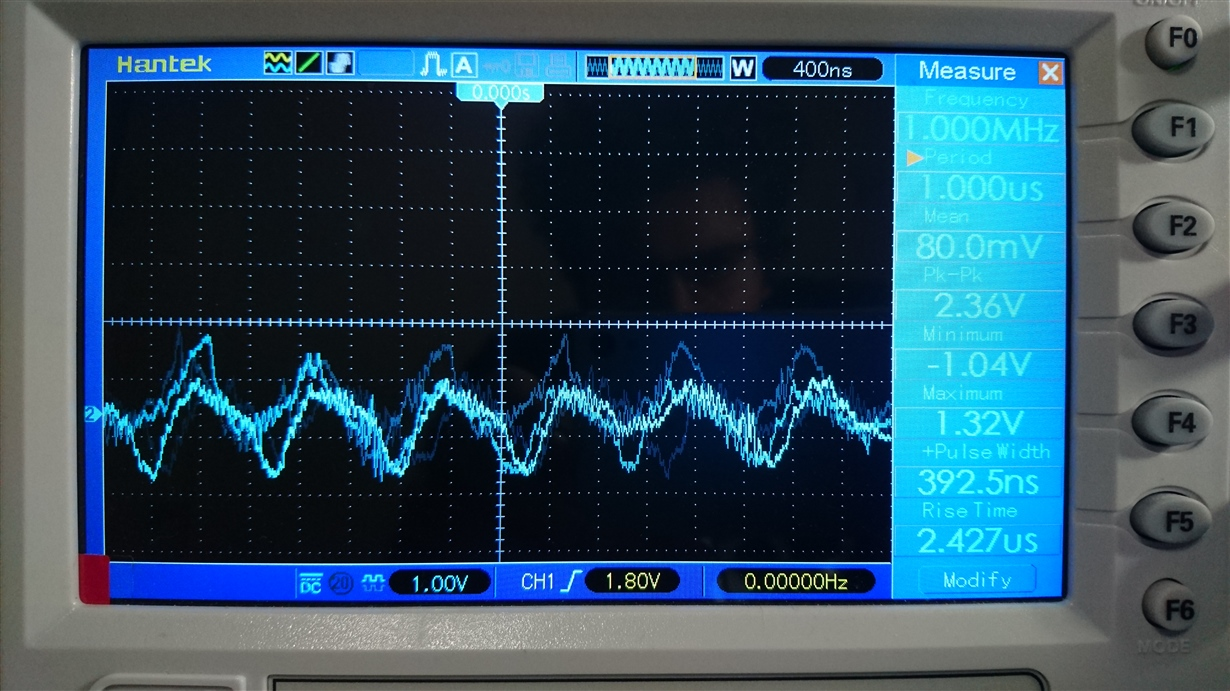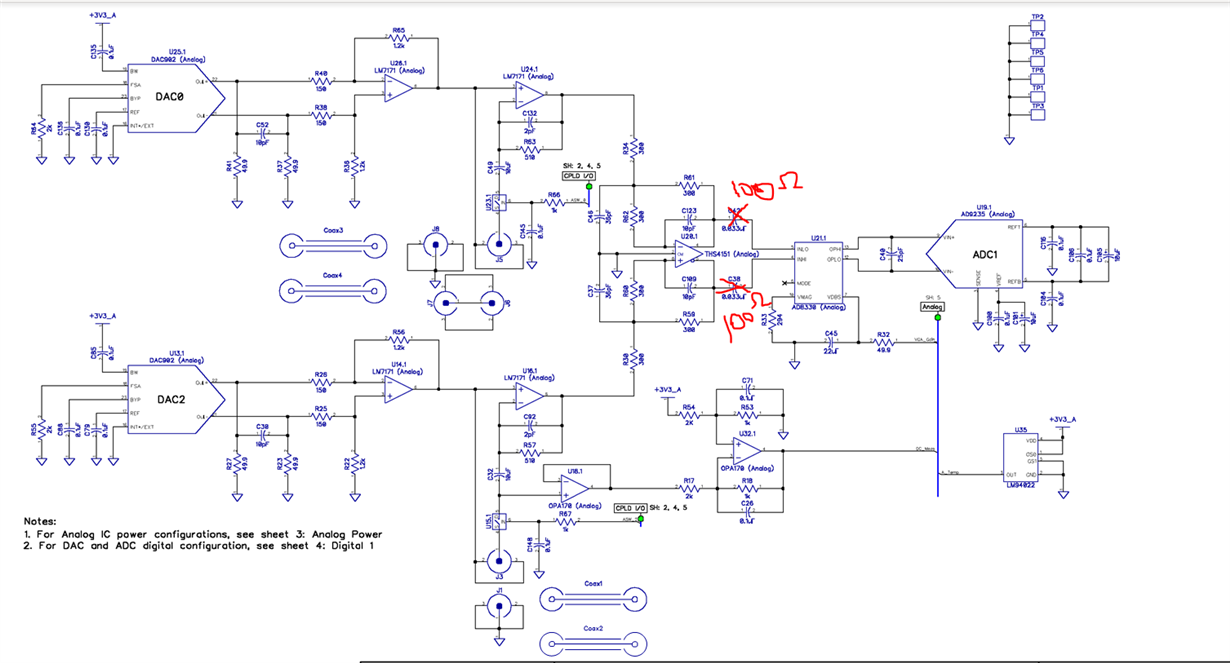# Op-amp Oscillation

Hi,

I have used the THS4151 op-amp to create a circuit that takes two inputs and subtract them to give an output. Below is the schematic for the circuit:R1 is where I will input a 1 MHz, 2V sinusoidal signal, and R6 is where the other input signal should come from. In this case, I grounded that input. Below is a picture of the input signal:My problem is when I try to power the op-amp, It will oscillate, and the input signal will look like:and the output look like this:

any suggestions?

Thank you

### 14 Replies

• well before someone runs a LG phase margin test, how are you measuring the output? Needs to be low capacitance sense.

Michael Steffes

Your circuit configuration is unstable, measuring at around 31 degrees of phase margin in sim. Not sure what your exact design requirements are, but if your goal is to subtract your inputs and achieve a differential output why not use the FDA in a typical configuration?

THS4151_Stability.TSC

Best,

Hasan Babiker

• In reply to Michael Steffes:

Hi,

I'm sorry for the late reply. Thank you for taking the time to reply to my post. Below I'm sharing the full schematics for what I'm working on:From the beginning, I was having trouble with the THS4151 it is over heating too. I think it's also effecting the other op-amps in the circuit. The output of the THS is two 100 ohms resistors which then it's connected to AD8330 VGA to gain the signal. Now, I did remove R34 and R30 and I did put in a 1 MHz sine wave from a function generator. I grounded the side of the R30 resistor so I know that it's 0 volts. This will allow to only worry on one side. Now, Normally, the two signals coming to R34 and R30 are the same unless the device is making a measurement in space, then the signal on the R34 side will be slightly higher. If there is any suggestion on how I can make the THS more stable would be appreciated.

Thank you

• In reply to Hasan Babiker31:

Hi,

I'm sorry for the late reply. Thank you for taking the time to reply to my post. Below I'm sharing the full schematics for what I'm working on:From the beginning, I was having trouble with the THS4151 it is over heating too. I think it's also effecting the other op-amps in the circuit. The output of the THS is two 100 ohms resistors which then it's connected to AD8330 VGA to gain the signal. Now, I did remove R34 and R30 and I did put in a 1 MHz sine wave from a function generator. I grounded the side of the R30 resistor so I know that it's 0 volts. This will allow to only worry on one side. Now, Normally, the two signals coming to R34 and R30 are the same unless the device is making a measurement in space, then the signal on the R34 side will be slightly higher. If there is any suggestion on how I can make the THS more stable would be appreciated.

Thank you

But when you are measuring that oscillation where and how are you making that measurement?

Michael Steffes

• In reply to Michael Steffes:

I'm not sure I understand your question correctly, but I'm measuring with the oscilloscope probe across the two 100 ohms resistors. I used a function generator to input the signal after removing R34 and R30 from the printed circuit board. The THS is very hot to the touch which tells me that it's oscillating without even measuring it.

Can you share your requirements for the THS4151 stage of your circuit? Gain, bandwidth, noise, etc.

Best,

Hasan Babiker

• In reply to Hasan Babiker31:

As far as the op amp bandwidth, I would be happy if I can get between 1-10 MHz. As far as the gain, it should be one. The signal will be gained using the AD8330 VGA. As far as the noise, I'm really not sure. As low as I can get it to be.

Thank you for taking the time to help me.